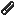Would you like to react to this message? Create an account in a few clicks or log in to continue.

# FORCE_STRAIN_GAUGE small variation

3 posters

#### DefranIn the attached schematic the gauge circuit has a very small variation, can you tell me how works this device in simulide, or may be it is a error?AttachmentsForce_Strain_Gauge.zip

Last edited by Defran on Sun May 21, 2023 11:01 pm; edited 1 time in total

#### Alex68#### arcachofoIn the attached schematic the gauge circuit has a very small variation, can you tell me how works this device in simulide, or may be it is a error?
Yes, it has a small variation, that's how it works.

I don't know much about the subject, but there are different ways to use it.
For example using Op. Amps. as Alex shows.
Or for example this:#### DefranMy quaestion was in order tu know about the dynamic range of this kind of sensors. have bought one and I have seen that in reality the range is also very narrow, around 60mv, so this is the one to work. Thanks.

Last edited by Defran on Tue Mar 07, 2023 11:00 pm; edited 2 times in total

#### arcachofoI can't give you much information because I didn't create this component.
Probably some parameter could be editable to match specific devices in the market, but I have little idea.
For example parameters about physical dimensions and maybe some other.

To get an idea of how it works here is the code that performs the calculation, from force in Newtons to resistance:

Code:
`// Parameters:double m_r0 = 120.0;double m_coef_poisson = 0.3;     // 0.27 - 0.34 Steel - Aludouble m_cste_bridgman = 1.3;    // around 1, 1.3 to get Constantan or Karma K=2.1double m_k_long;                 // gauge factor : perfect longitunal)double m_k;                      // gauge factor (include transverse decrease)double m_coef_transverse = 0.01; // to take into account non effectuve transverse wiresdouble m_ref_temp = 20.0;        // ambient reference for thermal driftdouble m_temp = 20.0;            // operating temperature (here no thermal drift)double m_young_modulus = 69e9;   // Aluminum test bodydouble m_h_body = 0.1e-3;        // rectangular section of test body , height=0.1 mmdouble m_w_body = 5e-3;          // width=5mmdouble m_section_body;double m_delta_r;                // resistance variation due to straindouble m_alpha_r  = 2e-5;        // per °C for Constantandouble m_lambda_j = 1.7e-5;      // per °C for Constantandouble m_lambda_s = 2.5e-5;      // per °C for Aluminum bodydouble m_delta_r_t;              // resisrance viariation due to thermal drift// Calculations:double Strain::sensorFunction( double forceN ){  double resisteance;  m_k_long = 1+2.0*m_coef_poisson+m_cste_bridgman*(1-2*m_coef_poisson);  m_k = m_k_long * (1 - m_coef_transverse);  m_section_body = m_h_body * m_w_body;  m_delta_r = m_r0*m_k*forceN/(m_young_modulus*m_section_body);  m_delta_r_t = (m_alpha_r+m_k*(m_lambda_s - m_lambda_j)) * (m_temp - m_ref_temp) * m_r0;  resisteance = m_r0 + m_delta_r + m_delta_r_t;  return resisteance;}`

#### DefranThanks, Arcacachofo. It is clear for mi now and also solved for the project in development.

arcachofo likes this post